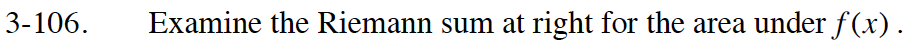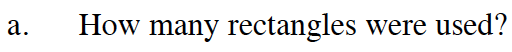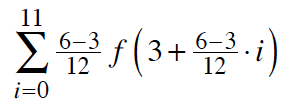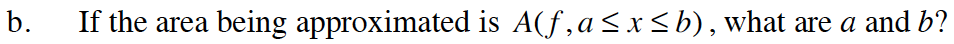### Home > CALC > Chapter Ch3 > Lesson 3.3.2 > Problem3-106

3-106.Notice that the index starts at 0 and ends at 11.

$\text{Also recall that }\Delta x= \frac{b-a}{n}, \text{ where }n \text{ represents number of rectangles.}$a = 3 is easy to spot in a Riemann sum. Now find the end-value, b.

General form of a left-endpoint Riemann sum:

$\sum_{i=0}^{n-1}\Delta xf(a+\Delta xi)$

You already computed how many rectangles there are part (a).

$\text{Notice that }\Delta x= \frac{6-3}{12}=\frac{1}{4}, \text{ so each rectangle has a width of }\frac{1}{4}.$